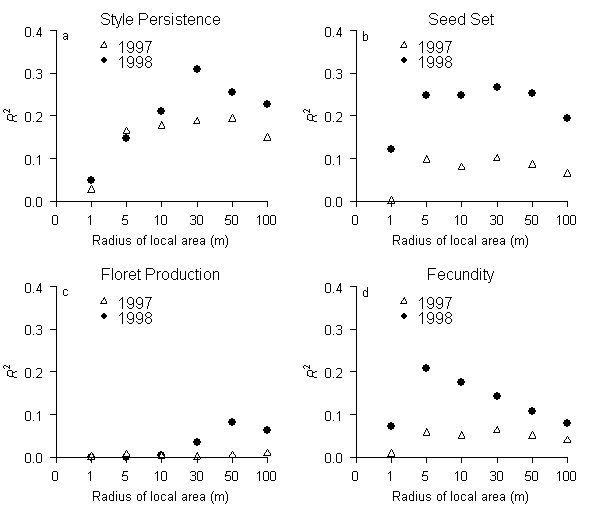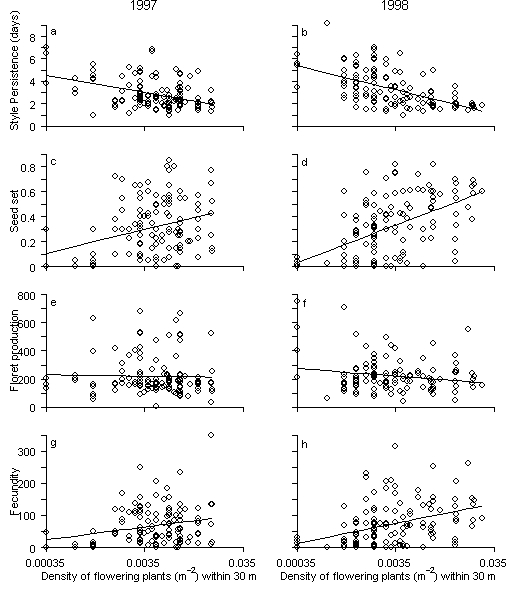Stuart Wagenius. 2006. Scale-dependence of reproductive failure in fragmented Echinacea populations. Ecology 87:931–941.

Appendix B. Figures showing the scale dependence of the relationship between individual-based Echinacea density and four individual annual reproductive fitness measures during two years.FIG. B1. Scale-dependent relationships between local density and four individual annual reproductive fitness measures over two years. R2 values for simple linear regressions between the density of flowering Echinacea plants within x meters and four fitness measures: (a) SP, style persistence in days, (b) the rate of seed set, (c) the number of florets per plant, and (d) the number of seeds produced. 1997: n = 118–127, 1998: n = 104–129.FIG. B2. The relationship between individual density within 30 m and four individual annual reproductive fitness measures (1997: n = 123, 1998: n = 127). Simple linear regressions are shown: (a) y = -0.6668* x – 0.753 4.546; r2 = 0.187, P < 0.000001; (b) y = -0.9303* x – 2.011; r2 = 0.310, P < 0.000001; (c) y = 0.08228* x + 0.7619; r2 = 0.100, P = 0.00029; (d) y = 0.1309* x + 1.0728; r2 = 0.267, P < 0.000001; (e) y = -3.989* x + 197.5; r2 = 0.0006, P = 0.780; (f) y = -23.43* x + 88.5; r2 = 0.035, P = 0.0393; (g) y = 17.35* x + 161.6; r2 = 0.062, P = 0.0047; (h) y = 26.74* x + 226.0; r2 = 0.142, P = 0.00002. Preserve plants in 1997 are not included in this graph because flowering Echinacea off of the transect were not mapped that year.

[Back to E087-054]